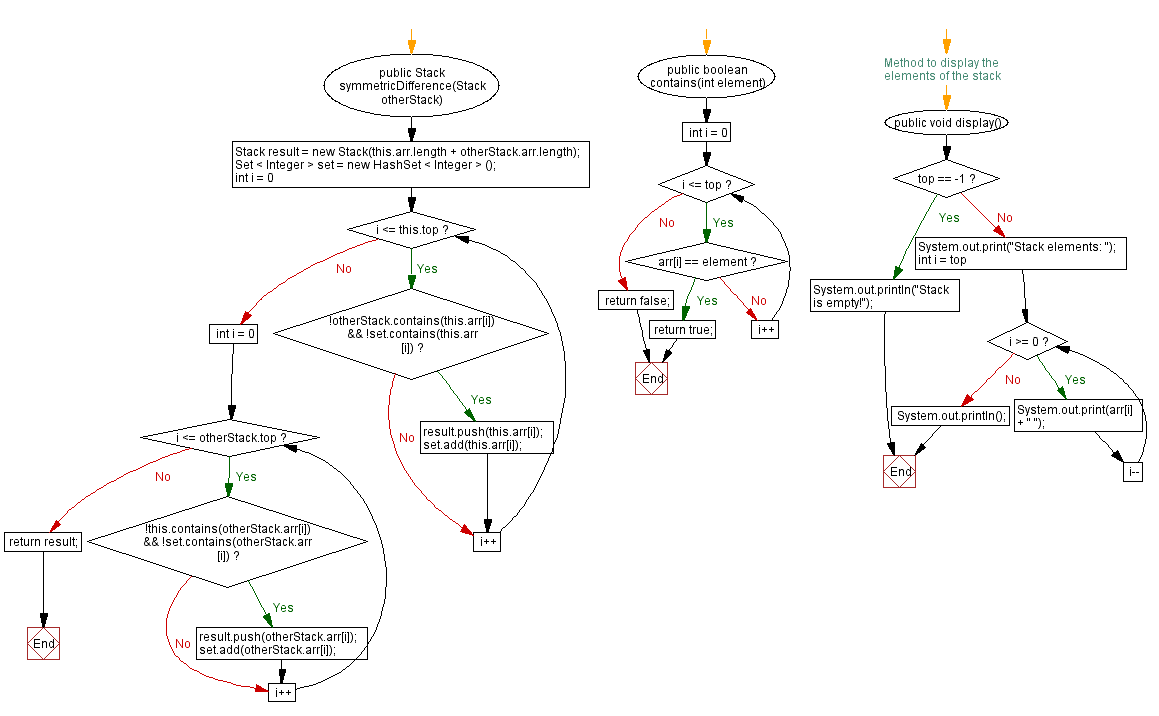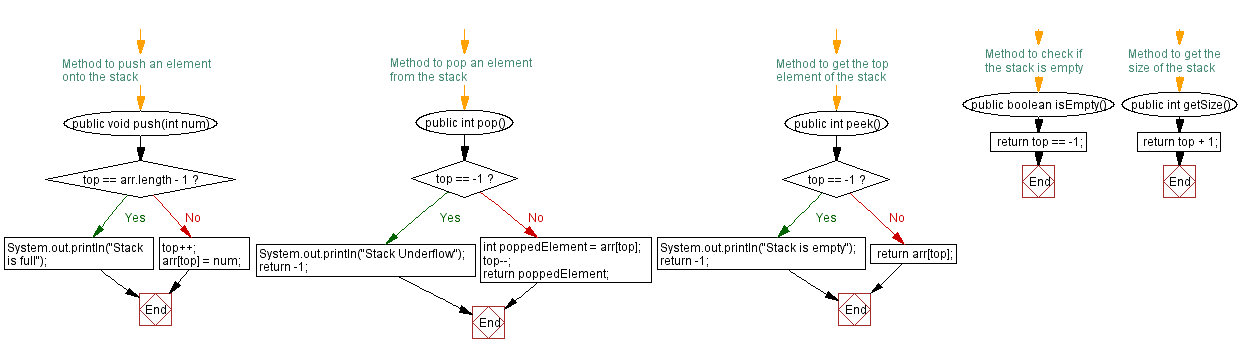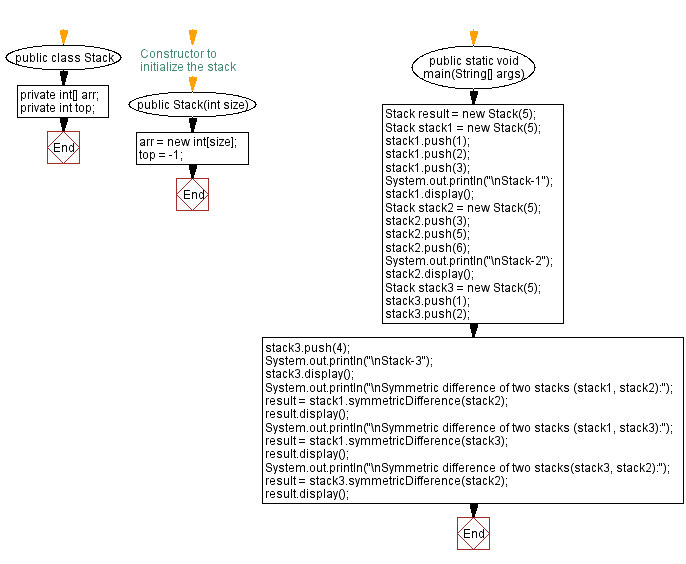# Java Exercises: Symmetric difference of two stacks

## Java Stack: Exercise-25 with Solution

In mathematics, the symmetric difference of two sets, also known as the disjunctive union, is the set of elements which are in either of the sets, but not in their intersection. For example, the symmetric difference of the sets [1,2,3} and {3,4} is {1,2,4}.

Write a Java program that implements a stack and creates a new stack that contains only elements that are in either the first or the second stack, but not in both.

Sample Solution:

Java Code:

``````import java.util.*;

public class Stack {
private int[] arr;
private int top;

// Constructor to initialize the stack
public Stack(int size) {
arr = new int[size];
top = -1;
}

// Method to push an element onto the stack
public void push(int num) {
if (top == arr.length - 1) {
System.out.println("Stack is full");
} else {
top++;
arr[top] = num;
}
}

// Method to pop an element from the stack
public int pop() {
if (top == -1) {
System.out.println("Stack Underflow");
return -1;
} else {
int poppedElement = arr[top];
top--;
return poppedElement;
}
}

// Method to get the top element of the stack
public int peek() {
if (top == -1) {
System.out.println("Stack is empty");
return -1;
} else {
return arr[top];
}
}

// Method to check if the stack is empty
public boolean isEmpty() {
}

// Method to get the size of the stack
public int getSize() {
}

public Stack symmetricDifference(Stack otherStack) {
Stack result = new Stack(this.arr.length + otherStack.arr.length);
Set < Integer > set = new HashSet < Integer > ();

for (int i = 0; i <= this.top; i++) {
if (!otherStack.contains(this.arr[i]) && !set.contains(this.arr[i])) {
result.push(this.arr[i]);
}
}

for (int i = 0; i <= otherStack.top; i++) {
if (!this.contains(otherStack.arr[i]) && !set.contains(otherStack.arr[i])) {
result.push(otherStack.arr[i]);
}
}

return result;
}

public boolean contains(int element) {
for (int i = 0; i <= top; i++) {
if (arr[i] == element) {
return true;
}
}
return false;
}

// Method to display the elements of the stack
public void display() {
if (top == -1) {
System.out.println("Stack is empty!");
} else {
System.out.print("Stack elements: ");
for (int i = top; i >= 0; i--) {
System.out.print(arr[i] + " ");
}
System.out.println();
}
}

public static void main(String[] args) {
Stack result = new Stack(5);
Stack stack1 = new Stack(5);
stack1.push(1);
stack1.push(2);
stack1.push(3);
System.out.println("\nStack-1");
stack1.display();
Stack stack2 = new Stack(5);
stack2.push(3);
stack2.push(5);
stack2.push(6);
System.out.println("\nStack-2");
stack2.display();
Stack stack3 = new Stack(5);
stack3.push(1);
stack3.push(2);
stack3.push(4);
System.out.println("\nStack-3");
stack3.display();
System.out.println("\nSymmetric difference of two stacks (stack1, stack2):");
result = stack1.symmetricDifference(stack2);
result.display();
System.out.println("\nSymmetric difference of two stacks (stack1, stack3):");
result = stack1.symmetricDifference(stack3);
result.display();
System.out.println("\nSymmetric difference of two stacks(stack3, stack2):");
result = stack3.symmetricDifference(stack2);
result.display();
}
}
```
```

Sample Output:

```Stack-1
Stack elements: 3 2 1

Stack-2
Stack elements: 6 5 3

Stack-3
Stack elements: 4 2 1

Symmetric difference of two stacks (stack1, stack2):
Stack elements: 6 5 2 1

Symmetric difference of two stacks (stack1, stack3):
Stack elements: 4 3

Symmetric difference of two stacks(stack3, stack2):
Stack elements: 6 5 3 4 2 1

```

Flowchart:Live Demo:

Java Code Editor:

Improve this sample solution and post your code through Disqus

Java Stack Previous: New stack from a portion of the original stack.
Java Stack Exercises Next: Verify all stack elements satisfy a condition.

What is the difficulty level of this exercise?

Test your Programming skills with w3resource's quiz.

﻿